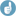## Precision in Endrényi L, Tóthfalusi L tables [Power / Sample Size]

Dear mittyri,

❝ here comes a magik (citing the paper):

The precision of the estimation was evaluated by running the simulations twenty times at twenty-two different conditions (different CV’s, different GMR’s and different designs).❝ Seed was sampled 'unfortunately' about 20 times?

What do you mean by 'unfortunately'?

This sentence describes an investigation of how precise the simulated power values are.
"The standard deviation of the simulated powers (20 values under each combination of CV, GMR and design, each with 10 000 sims; my insertion) was calculated under each condition; the mean of these standard deviations was 0.460. Thus, the precision of the power estimation is about ±0.5%."
They must have of course always choosen a different seed for the 20 repetitions under each combination of CV, GMR and design. Else you would get always the same result under each combination.

Of course they had also the opportunity to obtain a precision estimate via binomial distribution, without the burden of all that calculations. But then the random number generator had to be ideal.

BTW: Both Laszlos recommend to use PowerTOST if they were asked.

Regards,

DetlewIng. Helmut Schütz